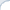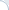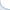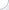The GNU C++ compiler produces efficient code for multiple platforms. The Intel compiler is specialized for Intel processors and produces even more efficient, but Intel-specific code. Yet, as some of us know, one does not get more than 10-20% improvement in most cases by switching from the GNU C++ compiler to the Intel compiler.
(The Intel compiler is free for non-commercial uses.)

There is at least one exception: programs that rely
heavily on a math library. It is not surprising that users of the Intel math library often enjoy almost a two-fold speedup over the GNU C++ library when they explicitly employ highly vectorized, linear algebra, and statistical functions. What is really amazing is that ordinary mathematical functions such as exp, cos, and sin can be computed 5-10 times faster by the Intel math library, apparently, without sacrificing accuracy.

Intel claims a 1-2 order-magnitude speedup for all standard math functions. To confirm this, I wrote simple benchmarks. They run on a modern Core i7 (3.4 GHz) processor in a single thread. The code (available from GitHub) generates random numbers that are used as arguments for various math functions. The intent of this is to represent plausible argument values. Intel also reports performance results for “working” argument intervals and admits that it can be quite expensive to compute functions (e.g., trigonometric) accurately for large argument values.

For single-precision numbers (i.e., floats), the GNU library is capable of computing only 30-100 million mathematical functions per second, while the Intel math library completes 400-1500 million of operations per second. For instance, it can do 600 million exponentiations or 400 million computations of the sine function (with single-precision arguments). This is slower than Intel claims, but still an order of magnitude faster than the GNU library.

Are there any accuracy tradeoffs? The Intel library can work in the high accuracy mode, in which, as Intel claims, the functions should have an error of at most 1 ULP (unit in the last place). Roughly speaking, the computed value may diverge from the exact one only in the last digit of mantissa. For the GNU math library, the errors are known to be as high as 2 ULP (e.g., for the cosine function with a double-precision argument). In the lowest accuracy mode, additional order-of-magnitude speedups are possible. It appears that the Intel math library should be superior to the GNU library in all respects. Note, however, that I did not verify Intel accuracy claims and I appreciate any links in regard to this topic. In my experiments, to ensure that the library works in the high-accuracy mode, I make a special call:

vmlSetMode(VML_HA);

I came across the problem of math-library efficiency, because I needed to perform many exponentiations to integer powers. There is a well-known approach called exponentiation by squaring and I hoped that the GNU math library implemented it efficiently. For example, to raise x to the power of 5, you first compute the square of x using one multiplication, and another multiplication to compute x4 (by squaring x2). Finally, you can multiply x4 by x and return the result. The total number of multiplications is three. Note that the naive algorithm would need four multiplications.

The function pow is overloaded, which means that there are several versions that serve arguments of different types. I wanted to ensure that the correct, i.e., the efficient version was called. Therefore, I told the compiler that the second argument is an unsigned (i.e., non-negative) integer as follows:

y = pow(x, (unsigned)5);

Big mistake! For the reason, which I cannot fully understand, this makes both compilers (Intel and GNU) choose an inefficient algorithm. As my benchmark shows (module testpow), it takes a modern Core i7 (3.4 GHz) processor almost 200 CPU cycles to compute x5. This is ridiculously slow, if you take into account that one multiplication can be done in one cycle (or less if we use SSE).

So, the following handcrafted implementation outperforms the standard pow by an order of magnitude (if the second argument is explicitly cast to unsigned):

float PowOptimPosExp0(float Base, unsigned Exp) {    if (!Exp) return 1;    float res = Base;    --Exp;     while (Exp) {        if (Exp & 1) res *= Base;         Base *= Base;        Exp >>= 1;    };     return res;};

If we remove the explicit cast to unsigned, the code is rather fast even with the GNU math library:

int IntegerDegree=5;pow(x, IntegerDegree);

Yet, my handcrafted function is still 20-50% faster than the GNU pow.

Turns out that it is also faster than the Intel's version. Can we make it even faster? One obvious reason for inefficiency are branches. Modern CPUs are gigantic conveyor belts that split a single operation into a sequence of dozens (if not hundreds) of micro operations. Branches may require the CPU to restart the conveyor, which is costly. In our case, it is beneficial to use only the forward branches. Each forward branch represents a single value of an integer exponent and contains the complete code to compute the function value. This code "knows" the exponent value and, thus, no additional branches are needed:

float PowOptimPosExp1(float Base, unsigned Exp) {    if (Exp == 0) return 1;    if (Exp == 1) return Base;    if (Exp == 2) return Base * Base;    if (Exp == 3) return Base * Base * Base;    if (Exp == 4) {        Base *= Base;        return Base * Base;    }     float res = Base;     if (Exp == 5) {        Base *= Base;        return res * Base * Base;    }     if (Exp == 6) {        Base *= Base;        res = Base;        Base *= Base;        return res * Base;    }     if (Exp == 7) {        Base *= Base;        res *= Base;        Base *= Base;        return res * Base;    }     if (Exp == 8) {        Base *= Base;        Base *= Base;        Base *= Base;        return Base;    }   …}

As a result, for the degrees 2-16, the Intel library performs 150-250 operations per second, while the customized version is capable of making 600-1200 exponentiations per second.

Acknowledgements: I thank Nathan Kurz and Daniel Lemire for the discussion and valuable links; Anna Belova for editing the entry.